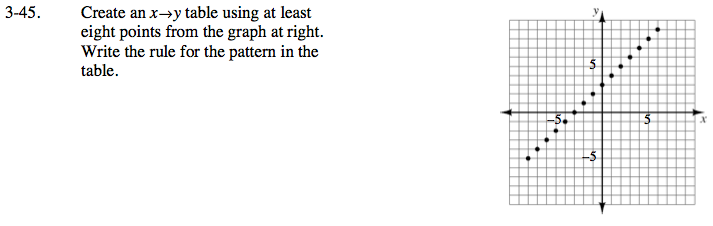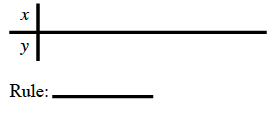### Home > CAAC > Chapter 3 > Lesson 3.1.5 > Problem3-45

3-45.Find a pattern in the points from the graph. Use the y = mx + b formula to find the rule.

Growth = m

The point b is the starting point, or where y = 0.

Rule: y = x + 3